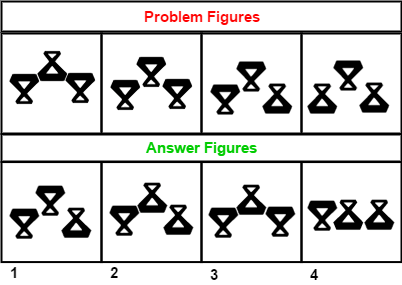# Non-Verbal Analogies - Logical Reasoning (MCQ) Questions for Q. 29961

Q.  The following question consists of some Problem Figures followed by other figures marked 1, 2, 3, 4 called the Answer Figures.

Find out the correct answer figure that should come next in the sequence of problem figures.- Published on 08 May 17

a. 1
b. 2
c. 3
d. 4

Starting from left to right, the figures are rotated by 180 degree (flipped vertically), one by one in a set order. The answer figure should be figure 1.

#### Discussion

• janardhan   -Posted on 22 Sep 22
sir if iam correct the answer is 3
• Abhishek   -Posted on 22 Sep 21
answer should be smaller part of all three upward
• Akarshan   -Posted on 23 Aug 20
the answer to this quetion is wrong plz correct it , the answer should be "smaller part of all three with upwards"

## ➨ Post your comment / Share knowledgeEnter the code shown above:

(Note: If you cannot read the numbers in the above image, reload the page to generate a new one.)